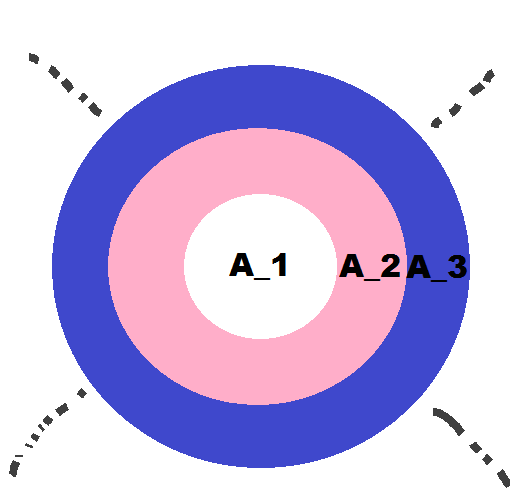# Inspired by toilet paper

Geometry Level 3Referring to the figure above, suppose $A_1$ is area of first white circle, then $A_2$ is area of pink annulus, $A_3$ is area of blue annulus and like wise $A_n$ would be the annulus bounded by $n^\text{th}$ and $(n-1)^\text{th}$ circle. These $n$ concentric circles are drawn such that the area bounded by two concentric circles remains constant, that is $A_1=A_2=A_3= \cdots = A_n$.

Define $r_n$ to be the radius of $n^\text{th}$ circle.Evaluate $\dfrac{r_{100}}{r_1}$ correct 2 decimal places.

×

Problem Loading...

Note Loading...

Set Loading...